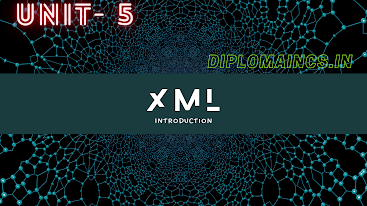## 1/4/20

### Previous year question for Computer Science and Engineering semester 4 Object Oriented Programming C++ year 2018

Object Oriented Programming C++ year 2018

Sub Code: - 1618404

2018(Even)
Time : 3Hrs
Semester  IV(New)
Object Oriented Programming C++

Full Marks : 70
Pass marks : 28

Group A

Choose the most suitable answer from the following options: -   (1*20=20)

(i) Which data type is used to represent the absence of parameter?

(a) int
(b) short
(c) void
(d) float

(ii) In C++ what is the sign of character type by default?

(a) Signed
(b) Unsigned
(c) Implementation dependent
(d) None of the above

(iii) The size of an
object or a type can be determined using which operator?
(a) malloc
(b) size of
(c) alloc
(d) calloc

(iv) Which of the following will not return a value?

(a) null
(b)  void
(c)  empty
(d)   free

(v) In which type does enumerators are stored by the compiler?

(a) string
(b) integer
(c) float
(d)  None of the above

(vi) The constants are also called as:

(a) Const.
(b) Preprocessor
(c) Literals
(d) None of the above

(vii) The data elements in structure are also known as what?

(a) Objects
(b) Member
(c) Data
(d) None of the above

(viii) What is this operator called?

(a) Conditional
(b) Relational
(c) Casting Operator
(d) None of the above

(ix) How many types of comments are there in C++?

(a) 1
(b) 2
(c) 3
(d) 4

(x)  Where does the execution of the program starts?

(a) User-defined function
(b) Main function
(c) Void function
(d) None of the above

(xi) How many minimum numbers of functions are need to be represented in C++?

(a) 0
(b) 1
(c) 2
(d) 3

(xii) In which of the following we cannot overload the function?

(a) Return function
(b) Caller
(c) Called function
(d) None of the above

(xiii) When our function need to return anything means what will we use/send as parameter in function?

(a)  void
(b)  Blank space
(c)  Both void and Blank space
(d) None of the above

(xiv) What does your class can hold?

(a) data
(b) functions
(c) Both data and functions
(d) None of the above

(xv) Where does the object is created?

(a) Class
(b) Constructor
(c) Destructor
(d) Attributes

(xvi) Pick the other name of operation function:

(d)  None of the above

(xvii) Which alternative can replace the throw statement?

(a)  for
(b) break
(c) return
(d) exit

(xviii) Where is the derived class is derived from:

(a) derived
(b) base
(c) both derived and base
(d) None of the above

(xix) Which symbol is used to create multiple inheritances?

(a) Dot
(b) Comma
(c) Dollar
(d) None of the above

(xx) Which header file is used to pass unknown number to arguments to function?

(a) stdlib.h
(b) string.h
(c) stdarg.h
(d) None of the above

Group:-"B"

Answer all Five Questions: -                         (5*4=20)

2. Explain the Const. Qualifier
OR

3. Difference between Constructor and Destructor
OR
Difference between structures and unions

OR
What do you mean by Virtual Function? Explain with examples.

5.  Define the following:
a.     Dynamic binding
b.     Polymorphism
c.      Encapsulation
OR
Define class and object? What is the relationship between them? Explain with examples.

6. Different between public, private and protected visibility modes.
OR
What is the importance of inline function? Explain with examples.

Group:- "C"

Answer all Five Questions: -                         (5*6=30)

7. Explain the various features of object oriented programming system in detail.
OR
Explain in detail about various types of control structures used in C++.

8. Write a program in C++ to arrange the elements of an array in ascending order.
OR
What are the different forms of inheritance? Give an example of each.

9. Explain various data types available in C++ using examples.
OR
Write a program in C++ to demonstrate single level inheritances.

10. Explain the concept of copy constructor by giving suitable examples.
OR
What is Polymorphism? Explain various types of polymorphism by giving suitable examples.

11.  Write down the relation between pointers and array in detail.
OR
Write short notes on the following:
a.      Static function
b.     Encapsulation

(Note:- Update available soon, comment for any type of help)

Data Structure using C: -Click me
Data base management system: - Click me
System Analysis and management information system- Click me

Semester 3rd previous year question

Computer Organization and Architecture  : - Click me
Operating System:-  Click me
Introduction to Software Package: - Click me
Computer Programing Through 'C':- Click me

Semester 5th previous year question
IWT - Software Engineering : - Click me
System maintenance: - Click me
Data Communication and Networking : - Click me
JAVA: - Click me